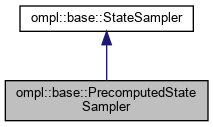ompl::base::PrecomputedStateSampler Class Reference

State space sampler for discrete states. More...

#include <ompl/base/PrecomputedStateSampler.h>

Inheritance diagram for ompl::base::PrecomputedStateSampler:[legend]

## Public Member Functions

PrecomputedStateSampler (const StateSpace *space, const std::vector< const State * > &states)
Constructor. Takes the state space to be sampled (space) and the set of states to draw samples from (states)

PrecomputedStateSampler (const StateSpace *space, const std::vector< const State * > &states, std::size_t minStateIndex, std::size_t maxStateIndex)
Constructor. Takes the state space to be sampled (space), the set of states to draw samples from (states) and a range to sample from: [minIndex, maxIndex].

void sampleUniform (State *state) override
Sample a state.

void sampleUniformNear (State *state, const State *near, double distance) override
Sample a state near another, within a neighborhood controlled by a distance parameter. More...

void sampleGaussian (State *state, const State *mean, double stdDev) override
Sample a state using a Gaussian distribution with given mean and standard deviation (stdDev). More...Public Member Functions inherited from ompl::base::StateSampler
StateSampler (const StateSampler &)=delete

StateSampleroperator= (const StateSampler &)=delete

StateSampler (const StateSpace *space)
Constructor.

## Protected Attributes

const std::vector< const State * > & states_
The states to sample from.

std::size_t minStateIndex_
The minimum index to start sampling at.

std::size_t maxStateIndex_
The maximum index to stop sampling at.Protected Attributes inherited from ompl::base::StateSampler
const StateSpacespace_
The state space this sampler samples.

RNG rng_
An instance of a random number generator.

## Detailed Description

State space sampler for discrete states.

Definition at line 45 of file PrecomputedStateSampler.h.

## ◆ sampleGaussian()

 void ompl::base::PrecomputedStateSampler::sampleGaussian ( State * state, const State * mean, double stdDev )
overridevirtual

Sample a state using a Gaussian distribution with given mean and standard deviation (stdDev).

As with sampleUniform, the implementation of sampleGaussian is specific to the derived class and few assumptions can be made about the distance between state and mean.

Implements ompl::base::StateSampler.

Definition at line 79 of file PrecomputedStateSampler.cpp.

## ◆ sampleUniformNear()

 void ompl::base::PrecomputedStateSampler::sampleUniformNear ( State * state, const State * near, double distance )
overridevirtual

Sample a state near another, within a neighborhood controlled by a distance parameter.

Typically, StateSampler-derived classes will return in state a state that is uniformly distributed within a ball with radius distance defined by the distance function from the corresponding state space. However, this is not guaranteed. For example, the default state sampler for the RealVectorStateSpace returns samples uniformly distributed using L_inf distance, while the default distance function is L_2 distance.

Implements ompl::base::StateSampler.

Definition at line 69 of file PrecomputedStateSampler.cpp.

The documentation for this class was generated from the following files: